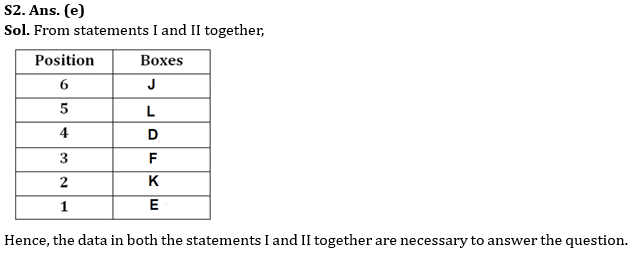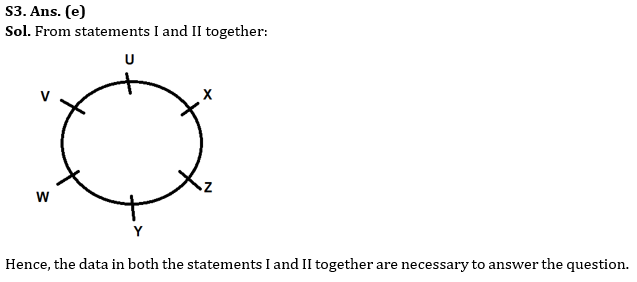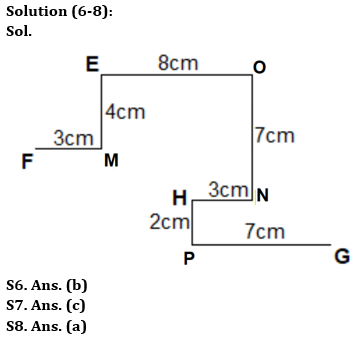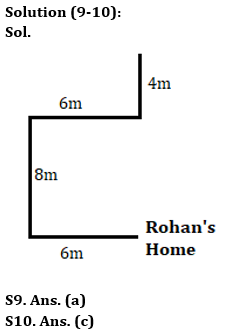Latest Banking jobs   »

# Reasoning Ability Quiz For Bank Foundation 2023 -11th May

Directions (1-5): The question given below is followed by two statements numbered I and II. You have to decide whether the data provided in the statements is sufficient to answer the question.

Q1. There are six persons namely, M to R of different ages. Who is the oldest person?
Statements:
I. Q is older than M who is older than O. R is younger than P.
Il. P is older than Q. N is not the oldest person.
(a) If the data in the statement I alone is sufficient to answer the question, while the data in statement II alone is not sufficient to answer the question.
(b) If the data in the statement II alone is sufficient to answer the question, while the data in statement I alone is not sufficient to answer the question.
(c) If the data either in the statement I alone or in statement II alone is sufficient to answer the question.
(d) If the data in both the statements I and II together are not sufficient to answer the question.
(e) If the data in both the statements I and II together are necessary to answer the question.

Q2. Six boxes, D, E, F, J, K and L are placed one above another but not necessarily in the same order, where the bottommost box is numbered as 1 and the box just above it is numbered as 2 and so on. Which box is placed at the topmost position?
Statements:
I. D is placed 2nd to the above of K which is placed just above of E. Two boxes are placed between K and L.
II. J is placed above D. F is not placed at the even numbered position.
(a) If the data in the statement I alone is sufficient to answer the question, while the data in statement II alone is not sufficient to answer the question.
(b) If the data in the statement II alone is sufficient to answer the question, while the data in statement I alone is not sufficient to answer the question.
(c) If the data either in the statement I alone or in statement II alone is sufficient to answer the question.
(d) If the data in both the statements I and II together are not sufficient to answer the question.
(e) If the data in both the statements I and II together are necessary to answer the question.

Q3. Six persons (W, X, Y, Z, U and V) are sitting around a circular table facing towards the center of the table but not necessarily in the same order. Who sits 2nd to the right of W?
Statements:
I. V sits adjacent to U. U sits 2nd to the left of W. X sits 2nd to the left of V.
II. U sits 2nd to the right of Z. Y sits adjacent to W but not to U.
(a) If the data in the statement I alone is sufficient to answer the question, while the data in statement II alone is not sufficient to answer the question.
(b) If the data in the statement II alone is sufficient to answer the question, while the data in statement I alone is not sufficient to answer the question.
(c) If the data either in the statement I alone or in statement II alone is sufficient to answer the question.
(d) If the data in both the statements I and II together are not sufficient to answer the question.
(e) If the data in both the statements I and II together are necessary to answer the question.

Q4. Eight boxes A, B, C, D, Q, R, S and T are kept one above another but not necessarily in the same order. The bottommost box is numbered as 1 and the topmost box is numbered as 8. How many boxes are kept between Box A and Box R?
Statements:
I. Only three boxes are kept below Box B. Three boxes are kept between Box B and Box T. Box R is kept at one of the places above Box N.
II. Only three boxes are kept between Box Q and Box R. Only one box is kept between Box R and Box D.
(a) If the data in the statement I alone is sufficient to answer the question, while the data in statement II alone is not sufficient to answer the question.
(b) If the data in the statement II alone is sufficient to answer the question, while the data in statement I alone is not sufficient to answer the question.
(c) If the data either in the statement I alone or in statement II alone is sufficient to answer the question.
(d) If the data in both the statements I and II together are not sufficient to answer the question.
(e) If the data in both the statements I and II together are necessary to answer the question.

Q5. Eight persons J, K, L, M, N, G, H, and I are born on 1st in the different months from January to August but not necessarily in the same order. Who is the youngest among them?
Statements:
I. Two persons born between N and G who was born immediately after I.
II. M was born before I but after H.
(a) If the data in the statement I alone is sufficient to answer the question, while the data in statement II alone is not sufficient to answer the question.
(b) If the data in the statement II alone is sufficient to answer the question, while the data in statement I alone is not sufficient to answer the question.
(c) If the data either in the statement I alone or in statement II alone is sufficient to answer the question.
(d) If the data in both the statements I and II together are not sufficient to answer the question.
(e) If the data in both the statements I and II together are necessary to answer the question.

Directions (6-8): Answer the questions based on the information given below:
Eight points are drawn on a sheet of paper such that F is 3cm west of M which is 4cm south of E. G is 7cm east of P which is 2cm south of H. O is 7cm north of N. E is 8cm west of O. N is 3cm east of H.

Q6. What is the shortest distance between E and G?
(a) 13cm
(b) 15cm
(c) 17cm
(d) 14cm
(e) None of these

Q7. In which direction is O with respect to H?
(a) North-west
(b) South-east
(c) North-east
(d) South-west
(e) None of these

Q8. In which direction is E with respect to N?
(a) North-west
(b) South-east
(c) North
(d) South-west
(e) None of these

Directions (9-10): Answer the questions based on the information given below.
Rohan starts walking from his home and walks in west direction for 6m, then turns right and walks for 8m. He again turns towards his right and walks for 6m and then turns towards his left and walks for 4m and then stops.

Q9. What is the total distance covered by Rohan?
(a) 24m
(b) 26m
(c) 32m
(d) 16m
(e) 48m

Q10. In which direction is Rohan’s final point with respect to his initial point?
(a) North-west
(b) South-east
(c) North
(d) South-west
(e) None of these

Solutions

Solution (1-5):
S1. Ans. (e)
Sol. From statements I and II together, we get P > Q > M > O, ___ > N and P > R
Hence, the data in both the statements I and II together are necessary to answer the question.S4. Ans. (d)
Sol. From statements I and II together, we get that the place of R is not certain. So, the data in both the statements I and II together are not necessary to answer the question.

S5. Ans. (d)
Sol. From statements I and II together, there is lack of information. So, the data in both the statements I and II together are not necessary to answer the question.## FAQs

### What is the selection process of the Bank Clerk?

The selection process of the Bank Clerk is Prelims & Mains.

#### Congratulations!Union Budget 2023-24: Free PDF